Ministério da Ciência, Tecnologia, Inovações e Comunicações CBPFIndex CENTRO BRASILEIRO DE PESQUISAS FÍSICAS12/08/2020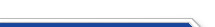Usuário: Senha: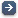Crie sua conta aqui · Busca rápida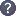Procurar por: Publicações Projetos EventosIr para a busca avançada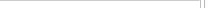Notas de Física
 From worldline to quantum superconformal mechanics with/without oscillatorial terms: $D(2,1;\alpha)$ and $sl(2|1)$ models Data do cadastro: 20/02/2017 Publicação/Divulgação: 20/02/2017 Resp. pelo cadastro: Status atual: Publicado Ano da publicação: 2016 Descrição: In this paper we quantize superconformal $\sigma$-models defined by worldline supermultiplets. \par Two types of superconformal mechanics, with and without a DFF term, are considered. \par Without a DFF term (Calogero potential only) the supersymmetry is unbroken. \par The models with a DFF term correspond to deformed (if the Calogero potential is present) or undeformed oscillators. For these (un)deformed oscillators the classical invariant superconformal algebra acts as a spectrum-generating algebra of the quantum theory.\par Besides the $osp(1|2)$ examples, we explicitly quantize the superconformally-invariant worldine $\sigma$-models defined by the ${\cal N}=4$ $(1,4,3)$ supermultiplet (with $D(2,1;\alpha)$ invariance, for $\alpha\neq 0,1$) and by the ${\cal N}=2$ $(2,2,0)$ supermultiplet (with two-dimensional target and $sl(2|1)$ invariance). The parameter $\alpha$ is the scaling dimension of the $(1,4,3)$ supermultiplet and, in the DFF case, has a direct interpretation as a vacuum energy. In the DFF case, for the $sl(2|1)$ models, the scaling dimension $\lambda$ is quantized (either $\lambda=\frac{1}{2}+{\mathbb Z}$ or $\lambda={\mathbb Z}$). The ordinary two-dimensional oscillator is recovered from $\lambda=-\frac{1}{2}$. The spectrum of the theory is decomposed into an infinite set of lowest weight representations of $sl(2|1)$. Surprisingly, extra fermionic raising operators, not belonging to $sl(2|1)$, allow to construct the whole spectrum from a single (for $\lambda=\frac{1}{2}+{\mathbb Z}$) bosonic vacuum. Número: CBPF-NF-006/16 Autores: I.E. Cunha; N.L. Holanda; F. Toppan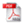Download do PDF (629 KB)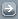Todos EventosTodos PublicaçõesTodasArtigos de Div. CientíficaArtigos em Revistas Nac.Artigos em Revistas Int.Artigos em Eventos Nac.Artigos em Eventos Int.Livros InteirosCapítulos de LivroPropriedades IntelectuaisÁudioPatentesProgramas de computadorModelos de utilidadeMarcasOutras prop. intel.TecnologiasKnow-howServiços tecnológicosTecnologias inovadorasTeses de DoutoradoDissertações de MestradoProjetos de GraduaçãoCiência e SociedadeDocumentos HistóricosMonografiasNotas de FísicaNotas TécnicasOutras Produções
 · Principal    |    CBPF    |    · Copyright 2004 - CBPF. Rio de Janeiro/BrasilCentro Brasileiro de Pesquisas Físicasv 1.1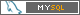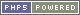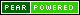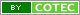Pág. gerada em 0.01 s Total de consultas SQL: 26